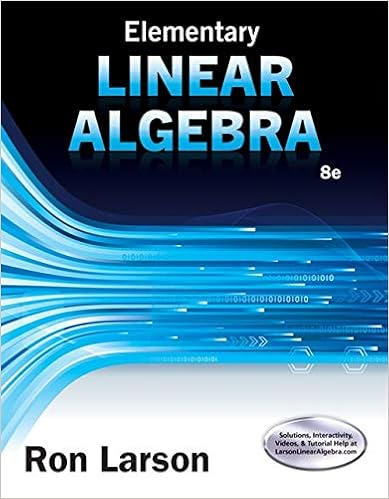# Another sensible choice for a basis of im a is e 1 e

• Notes
• 56
• 100% (1) 1 out of 1 people found this document helpful

This preview shows page 15 - 18 out of 56 pages.

##### We have textbook solutions for you!
The document you are viewing contains questions related to this textbook.The document you are viewing contains questions related to this textbook.
Chapter 6 / Exercise 36
Elementary Linear Algebra
LarsonExpert Verified
Another sensible choice for a basis of im( A ) is e 1 , e 2 , e 3 . 29. The three column vectors of A span all of R 2 , so that im( A ) = R 2 . We can choose any two of the columns of A to form a basis of im( A ); another sensible choice is e 1 , e 2 . 30. im( A ) = span( e 1 , e 2 ) We can choose e 1 , e 2 as a basis of im( A ). 31. The two column vectors of the given matrix A are linearly independent (they are not parallel), so that they form a basis of im( A ). 137
##### We have textbook solutions for you!
The document you are viewing contains questions related to this textbook.The document you are viewing contains questions related to this textbook.
Chapter 6 / Exercise 36
Elementary Linear Algebra
LarsonExpert Verified
Chapter 3 ISM: Linear Algebra 32. By inspection, the first, third and sixth columns are redundant. Thus, a basis of the image consists of the remaining column vectors: 1 0 0 0 , 0 1 0 0 , 0 0 1 0 . 33. im( A ) = span( e 1 , e 2 , e 3 ), so that e 1 , e 2 , e 3 is a basis of im( A ). 34. The fact that 1 2 3 4 is in ker( A ) means that A 1 2 3 4 = [ v 1 v 2 v 3 v 4 ] 1 2 3 4 = v 1 + 2 v 2 + 3 v 3 + 4 v 4 = 0, so that v 4 = - 1 4 v 1 - 1 2 v 2 - 3 4 v 3 . 35. If v i is a linear combination of the other vectors in the list, v i = c 1 v 1 + · · · + c i - 1 v i - 1 + c i +1 v i +1 + · · · + c n v n , then we can subtract v i from both sides to generate a nontrivial relation (the coe ffi cient of v i will be -1). Conversely, if there is a nontrivial relation c 1 v 1 + · · · + c i v i + · · · + c n v n = 0, with c i = 0, then we can solve for vector v i and thus express v i as a linear combination of the other vectors in the list. 36. Yes; we know that there is a nontrivial relation c 1 v 1 + c 2 v 2 + · · · + c m v m = 0. Now apply the transformation T to the vectors on both sides, and use linearity: T ( c 1 v 1 + c 2 v 2 + · · · + c m v m ) = T (0), so that c 1 T ( v 1 ) + c 2 T ( v 2 ) + · · · + c m T ( v m ) = 0. This is a nontrivial relation among the vectors T ( v 1 ) , . . . , T ( v m ), so that these vectors are linearly dependent, as claimed. 37. No; as a counterexample, consider the extreme case when T is the zero transformation, that is, T ( x ) = 0 for all x . Then the vectors T ( v 1 ) , . . . , T ( v m ) will all be zero, so that they are linearly dependent. 38. a. Using the terminology introduced in the exercise, we need to show that any vector v in V is a linear combination of v 1 , . . . , v m . Choose a specific vector v in V . Since we can find no more than m linearly independent vectors in V , the m + 1 vectors v 1 , . . . , v m , v will be linearly dependent. Since the vectors v 1 , . . . , v m are independent, v must be redundant, meaning that v is a linear combination of v 1 , . . . , v m , as claimed. 138
ISM: Linear Algebra Section 3.2 b. With the terminology introduced in part a, we can let V = im [ v 1 · · · v m ] . 39. Yes; the vectors are linearly independent. The vectors in the list v 1 , . . . , v m are linearly independent (and therefore non-redundant), and v is non-redundant since it fails to be in the span of v 1 , . . . , v m . 40. Yes; by Fact 3.2.8, ker( A ) = { 0 } and ker( B ) = { 0 } . Then ker( AB ) = { 0 } by Exer- cise 3.1.51, so that the columns of AB are linearly independent, by Fact 3.2.8.
•••